# Prof. Goodman's solution

## Math 222, Fall 2021

### Introduction:

In this assignment you will apply numerical methods method to solve(1)
from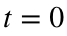to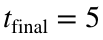.
Note that this problem has an exact solution:(2).
Assignment instructions:
• Before you get started, replace the words your_name in the file name and in the title with your actual name.
• In each of the code blocks, you may cut and paste code from the example given in the assignment or else write your own.
• Any plots should be meaningfully labeled and titled.
• When you finish the assignment, run this live script. When all the outputs are correct and the plots look good, click on the Export in the menu at the top and save as a PDF.
• Turn in the PDF on Canvas.

## Question 1

### Part 1

In this part, you will solve the ODE (1) using Euler's method and reduce the error by increasing the number of time steps, N.
Task 1. Let N be the number of time steps, so that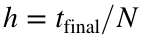. At first, we'll just take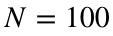, but we will modify that later. Write code to solve the ODE using the Euler method. Define your ODE function at the bottom of the script. Store the solution in a vector, y.
Prof Goodman notes: I found that I needed N=450 points to get my error below 0.01. That seems like a lot to me.
tFinal = 5;
N = 450;
h=tFinal/N;
t=linspace(0,tFinal,N+1); % type 'help linspace' to see how this works
y=zeros(1,N+1);
y(1) = 1/3; % setting the initial condition
for n=1:N
y(n+1) = y(n) + h * f(t(n),y(n));
end
Task 2. Define a function exact_solution at the bottom of the fileand use it to compute the exact solution to the ODE. Store the output in a vector called y_exact.
yExact=exact_solution(t);
Task 3. Plot the numerically estimated solution and the exact solution on one axis.
plot(t,y,t,yExact,'--');
xlabel('t'); ylabel('y');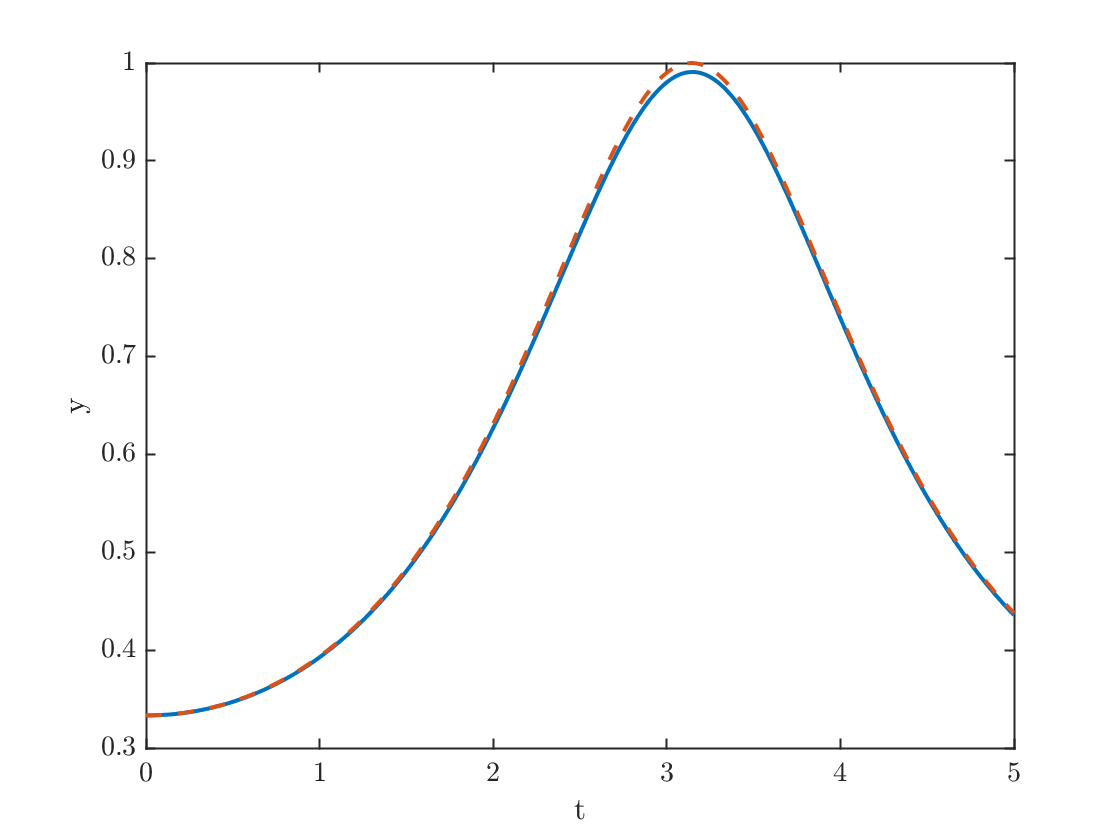Task 4. Plot the error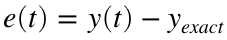as a function of t.
plot(t,y-yExact)
xlabel('t')
ylabel('error in y')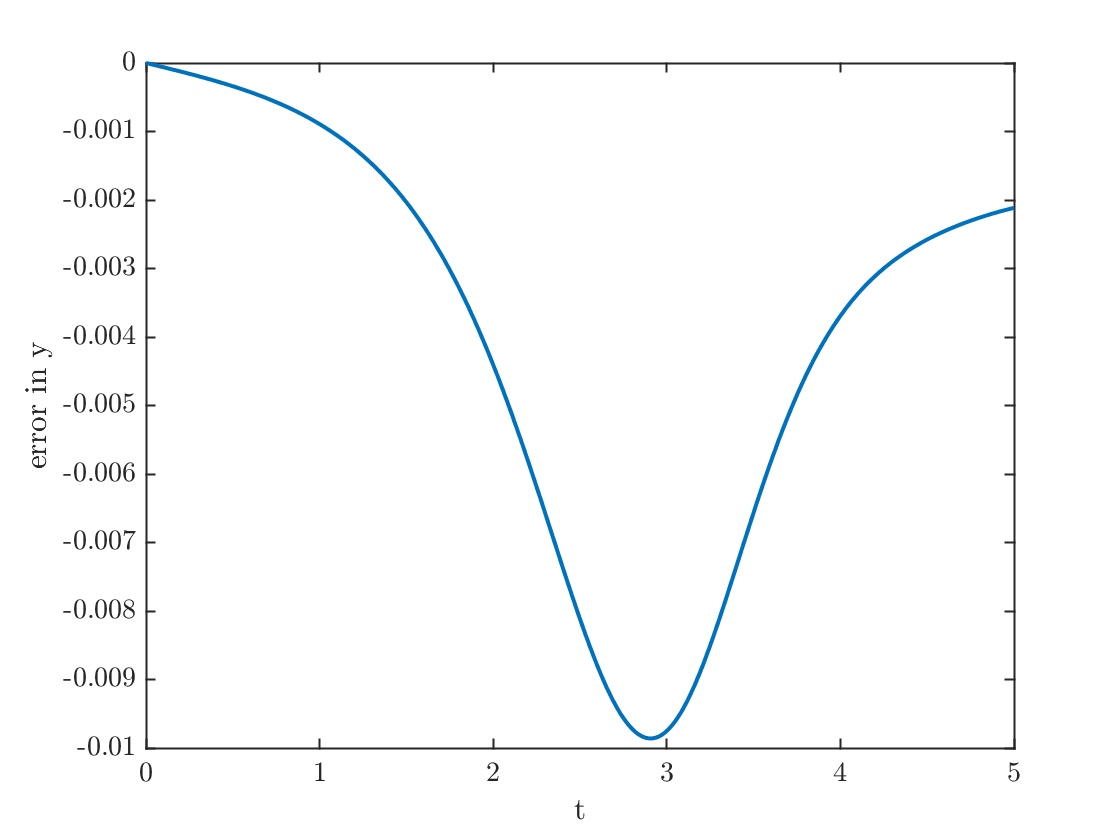Task 5. Compute the maximum of the absolute value error between y and y_exact as: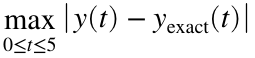Store your result in a variable named error1.
error1=max(abs(y-yExact));
fprintf('The maximum error is %0.4f.\n',error1)
The maximum error is 0.0099.
Task 6. Figure out what is the minimum value of N that you need to get the maximum error smaller than 0.01, i.e find N so that: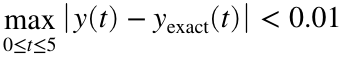• Experiment with N in Task 1 until you get a small enough error. Make sure you use the minimum value of N that produces a small enough error.
• You can compute the maximum of an array using the max function with the syntax: [xmax,p]=max(x);. In this case xmax will be the maximum value of the vector x and p will be the index where it is obtained, i.e., xmax=x(p). For more information, see the documentation for the max function.

### Part 2

In this part, you will show that Euler's method is approximately first order.
Task 1. Copy your code from Task 1 of the previous part, but edit the code to use double the value of N you used previously.
N = 900;
h=tFinal/N;
t=linspace(0,tFinal,N+1); % type 'help linspace' to see how this works
y=zeros(1,N+1);
y(1) = 1/3; % setting the initial condition
for n=1:N
y(n+1) = y(n) + h * f(t(n),y(n));
end
Task 2. Compute y_exact using the new t vector you just computed. Use this to compute the maximum of the absolute value error between y and y_exact as:Store your result in error2, and display it.
yExact=exact_solution(t);
error2=max(abs(y-yExact));
Task 3. Display error1/error2 to show that the error has been reduced by about a factor of two.
fprintf('The maximum error went down by a factor of %f.\n',error1/error2);
The maximum error went down by a factor of 1.982089.

## Question 2

• Implement the improved Euler method described in the assignment.
• Find the minimum value of N needed to get the maximum error below 0.01

### Part 1

Repeat the steps given in Part 1 of Question 1. First, successfuly implement the improved Euler method, and, second, experiment with the value of N needed to get the error small enough.
N=31;
h=tFinal/N;
t=linspace(0,tFinal,N+1);
y=zeros(1,N+1);
y(1)=1/3;
for n=1:N
k1 = f(t(n),y(n));
k2 = f(t(n)+h, y(n)+h*k1);
y(n+1) = y(n) + h * (k1+k2)/2;
end
yExact=exact_solution(t);
plot(t,y,t,yExact,'--');
xlabel('t');ylabel('y');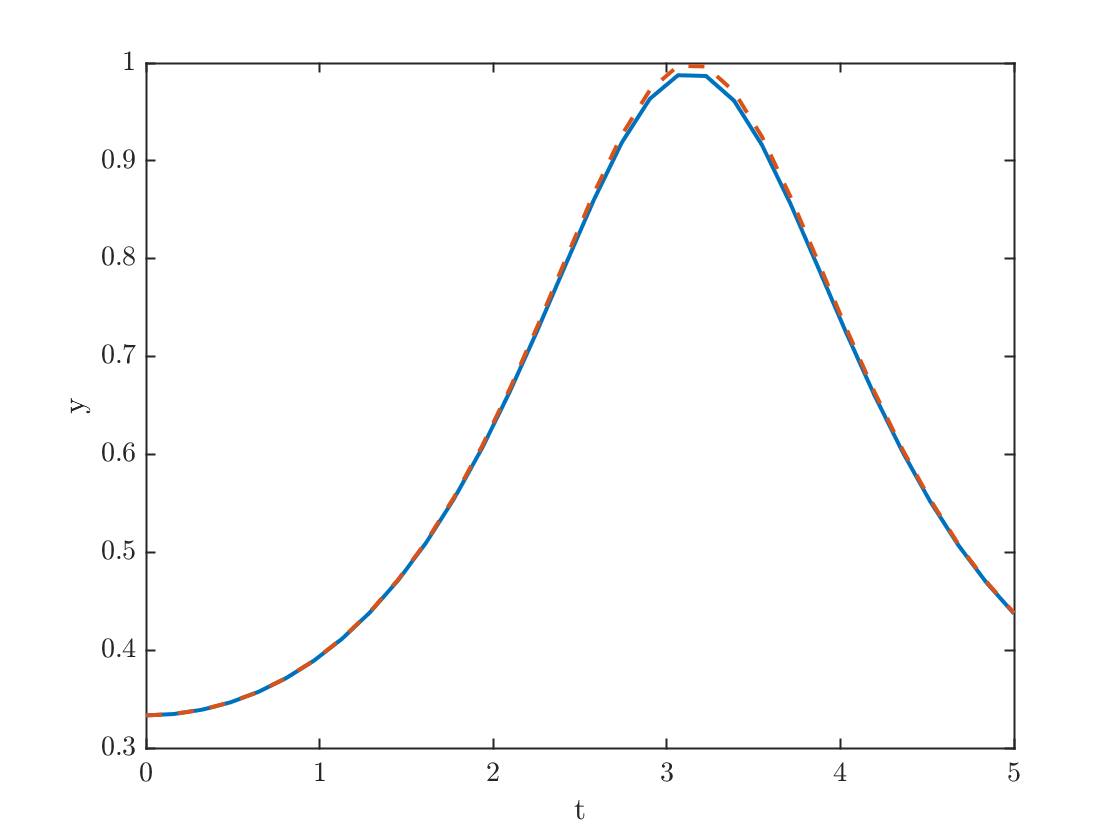error1=max(abs(y-yExact));
fprintf('Maximum error is %0.4f.\n',error1)
Maximum error is 0.0097.

### Part 2

Repeat the steps given in Part 2 of Question 1, i.e., double the number of points and see the error goes down by a factor of four.
N=62;
h=tFinal/N;
t=linspace(0,tFinal,N+1);
y=zeros(1,N+1);
y(1)=1/3;
for n=1:N
k1 = f(t(n),y(n));
k2 = f(t(n)+h, y(n)+h*k1);
y(n+1) = y(n) + h * (k1+k2)/2;
end
yExact=exact_solution(t);
error2=max(abs(y-yExact));
fprintf('The maximum error went down by a factor of %f.\n',error1/error2);
The maximum error went down by a factor of 4.030093.

## Answer the following questions right in the livescript

The Euler method taught in the book is used mainly in teaching. In practice, people writing simulations are likely to use second order methods like improved Euler, or even higher order methods. The most commonly used method is fourth order. Explain why you think this is important.
It was really impressive that we got the same error with only 31 steps of the improved method as we did with 450 steps of the Euler method. Even though each step took twice as many function evaluations per step for a total of 62 function evaluations, which means we got the same accuracy with about 14% as much work. If we needed more digits of accuracy, we would save even more. Matlab's most popular ODE solver is fourth order, and this year they introduced at 8th order method. Think how much accuracy you need to land a probe on Mars. You simply couldn't do that if you used the Euler method.
What did you learn doing this assignment?
I can't answer that for the students. (Graders, give them points if they say something thoughtful.)
What was confusing about the assignment that might have been explained better?
I think I should have explained those fprintf statements that I included. I bet those were confusing. (Graders, give them points if they say something thoughtful.)

## Functions used

function yprime=f(t,y)
% Define the function that forms the right-hand side of the ODE
yprime = sin(t)*y^2;
end
function y=exact_solution(t)
% Define the exact solution
y = 1./(2+cos(t));
end